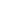H-129, Palri, Hodal, Palwal, HR
+91 9992755268

# How to create FizzBuzz in Javascript

FizzBuzz in js is a basic program to make the beginners better understanding of their own code. using this you can learn how the code actually works. here are two methods in javascript to create FizzBuzz.

Table of Content - Learn PHP Online
1. Using the Function
2. using for Loop

Q: Write a program that uses console.log to print all the numbers from 1 to 100,
with two exceptions. For numbers divisible by 3, print “Fizz” instead of the number,
and for numbers divisible by 5 (and not 3), print “Buzz” instead.
When you have that working, modify your program to print “FizzBuzz”,
for numbers that are divisible by both 3 and 5 (and still print “Fizz” or “Buzz” for numbers divisible by only one of those).
(This is actually an interview question that has been claimed to weed out a significant percentage of programmer candidates. So if you solved it, you’re now allowed to feel good about yourself.)

##### Let’s starting writing the code here ” How to create FizzBuzz in js” using Function

using the function method is best way here we can set the time interval between first step and second step. and I think you can easily understand the code and use it for yourself.

```<script>
var a,b,c;  // intialize three variables
a=0;
b=0;
c=0;

function demo(){
a++;

if( a % 15 == 0 )
{
b='fizzBuzz';
document.write(b + "<br>");
}
else if( a % 3 == 0 )
{
b='fizz';
document.write(b + "<br>");
}
else if( a%5==0 )
{
c='Buzz';
document.write(c + "<br>");
}
else{
document.write(a +"<br>");
}

// stop set interval after 100

if(a==100)
{
clearInterval(start);
}

}

// setInterval repeat calling any function infinite times
var start =setInterval(demo,500);
</script>
```
##### 2.Now the Second method  ” How to create FizzBuzz in js” using For Loop
```<script>

for (var i=1; i <= 100; i++)
{
if (i % 15 == 0)
document.write("FizzBuzz" + "<br>");
else if (i % 3 == 0)
document.write("Fizz" + "<br>");
else if (i % 5 == 0)
document.write("Buzz" + "<br>");
else
document.write(i +"<br>");
}

</script>```

Summary

“How to create FizzBuzz in js”: Now you knows the program works in many ways and  its totally depends on programmer. FizzBuzz is the best way to understand conditions.